Case Based Questions (MCQ)

Class 10
Chapter 10 Class 10 - Light - Reflection and Refraction

## A student focuses the image of a candle flame, placed at about 2 m from a convex lens of focal length 10 cm, on a screen. After that he gradually moves the flame towards the lens and each time focuses its image on the screen.

### (iv) away from flame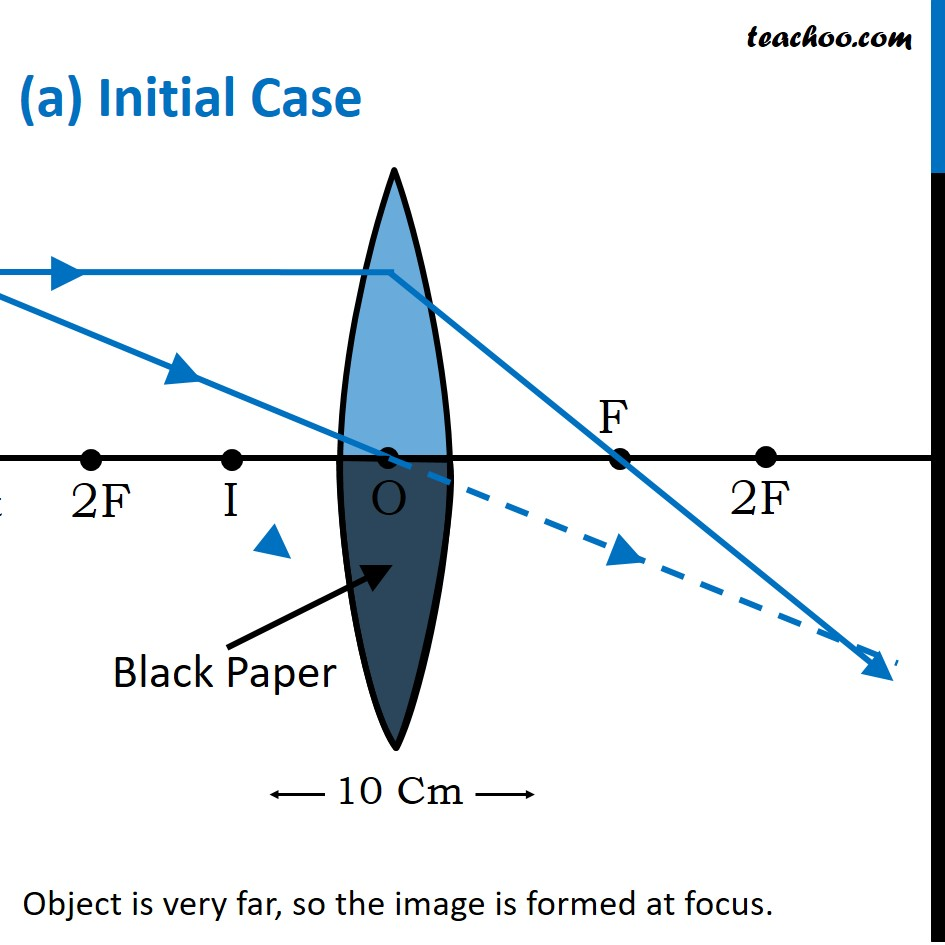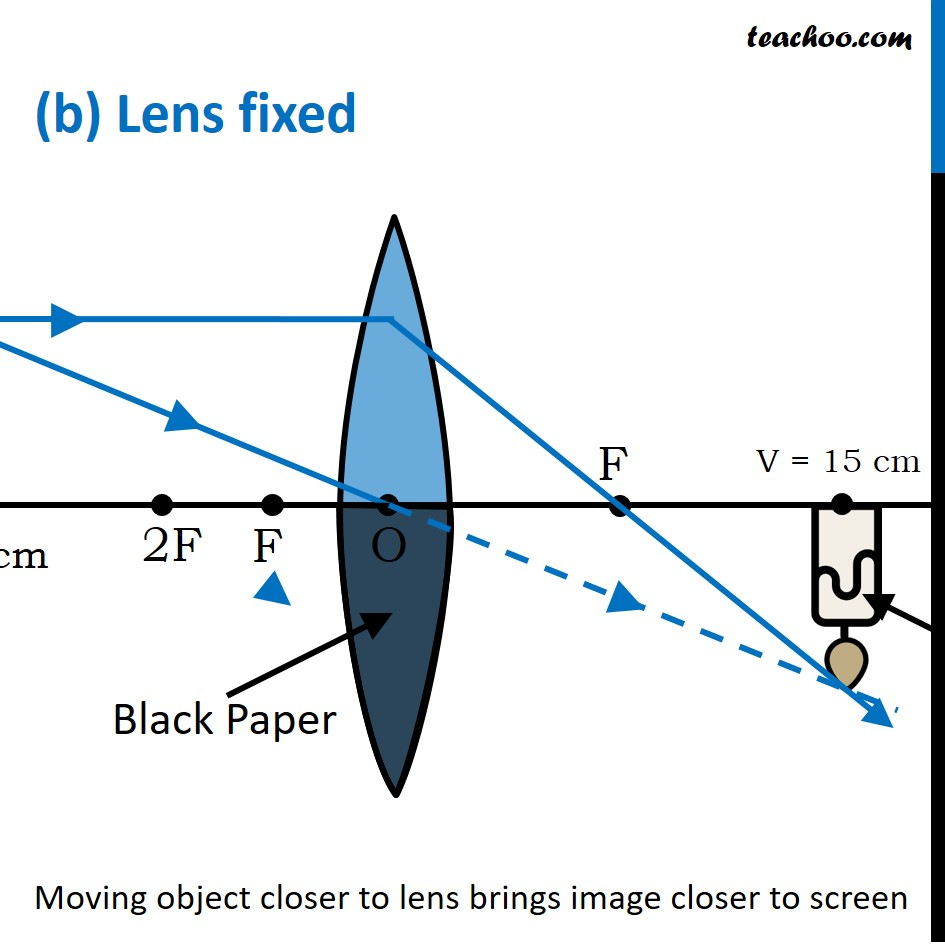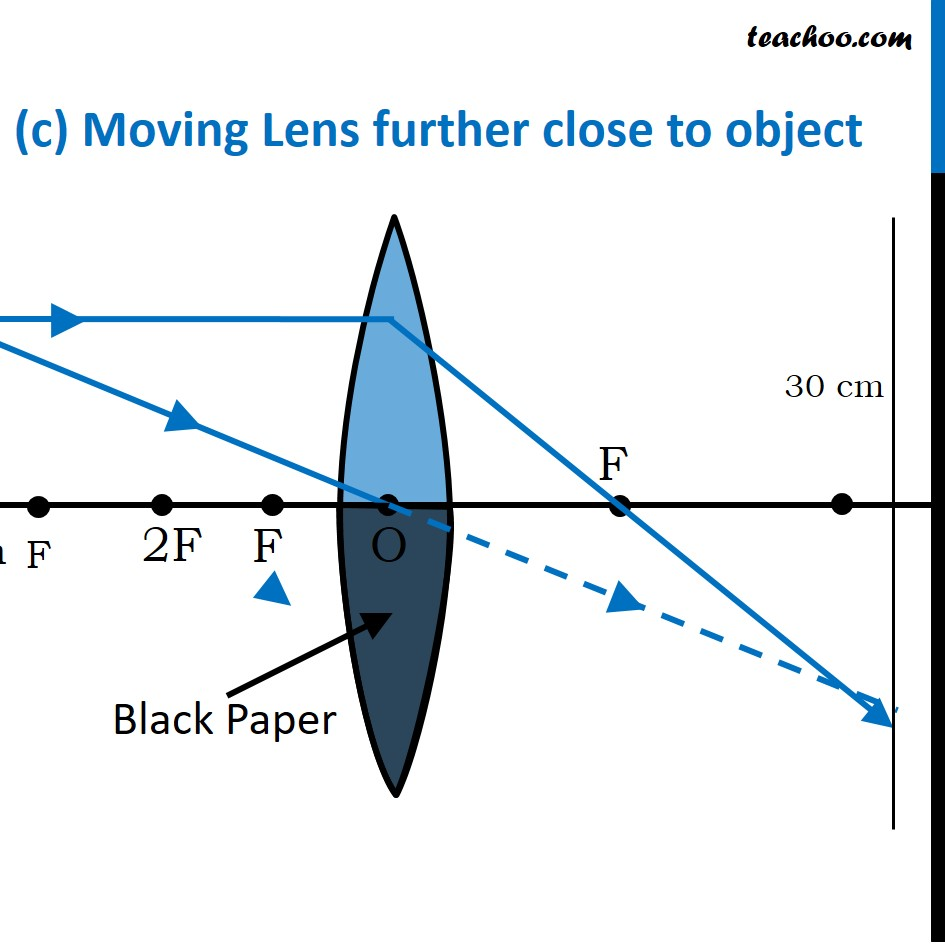In a convex lens

As the object and lens come closer (‘u’ decreases) , the image distance (‘v’) increases.

Let us check

• Moving lens away from screen
• Choosing a point much closer to the lens, let’s say 30 cm (beyond 2F), we see the image move closer to the screen, but still not properly focused.
• Now, we move the lens further away from the screen (close to the objects), and away from the screen (let’s say at 15 cm, b/w C and F), we can see the proper image forming on the screen (beyond 2F)
• To move the object closer to the lens, the lens must be moved away from the screen so the image can be focused.
• Moving towards the screen, the image to form closer to the lens, resulting in blurring
• If we do not move , we will see no change in the image.
• Moving the lens away from the flame takes us closer to the screen (same as option (ii))

Thus, the correct answer is (i) away from screen

### (iv) size will become too small

Ans.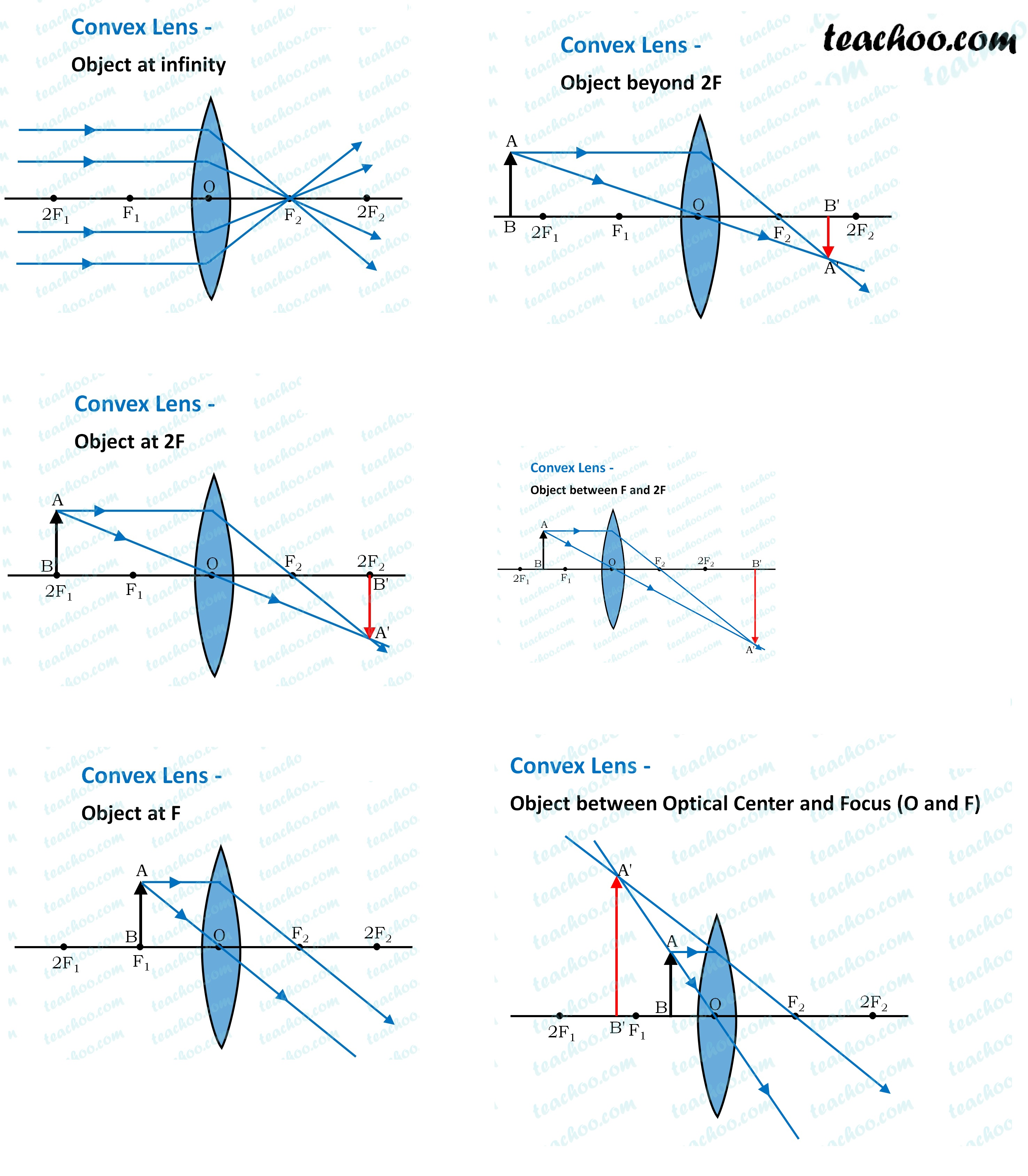In a convex lens

• As the object moves towards the lens, the size of the image increases
• until the object crosses the focus.
• Between F and P, the image becomes virtual.

Thus, the correct answer is (ii) size of image will increase

### (iv) the flame disappears

Ans.

Intensity is the amount of energy per unit area per unit time .

Thus

• Intensity is inversely proportional to area
• As we move closer, the size of the image increases
• As size increases, area increases
• Thus, intensity decreases

Thus, the correct answer is (iii) intensity of flame reduces

### (iv)no image

Ans.

Now,

• Given focal length of convex lens is 10 cm
• Thus, 5 cm means that object is between F and P.
• Convex lens produces a virtual image when object is between F and P .
• Hence, this image cannot be seen on screen.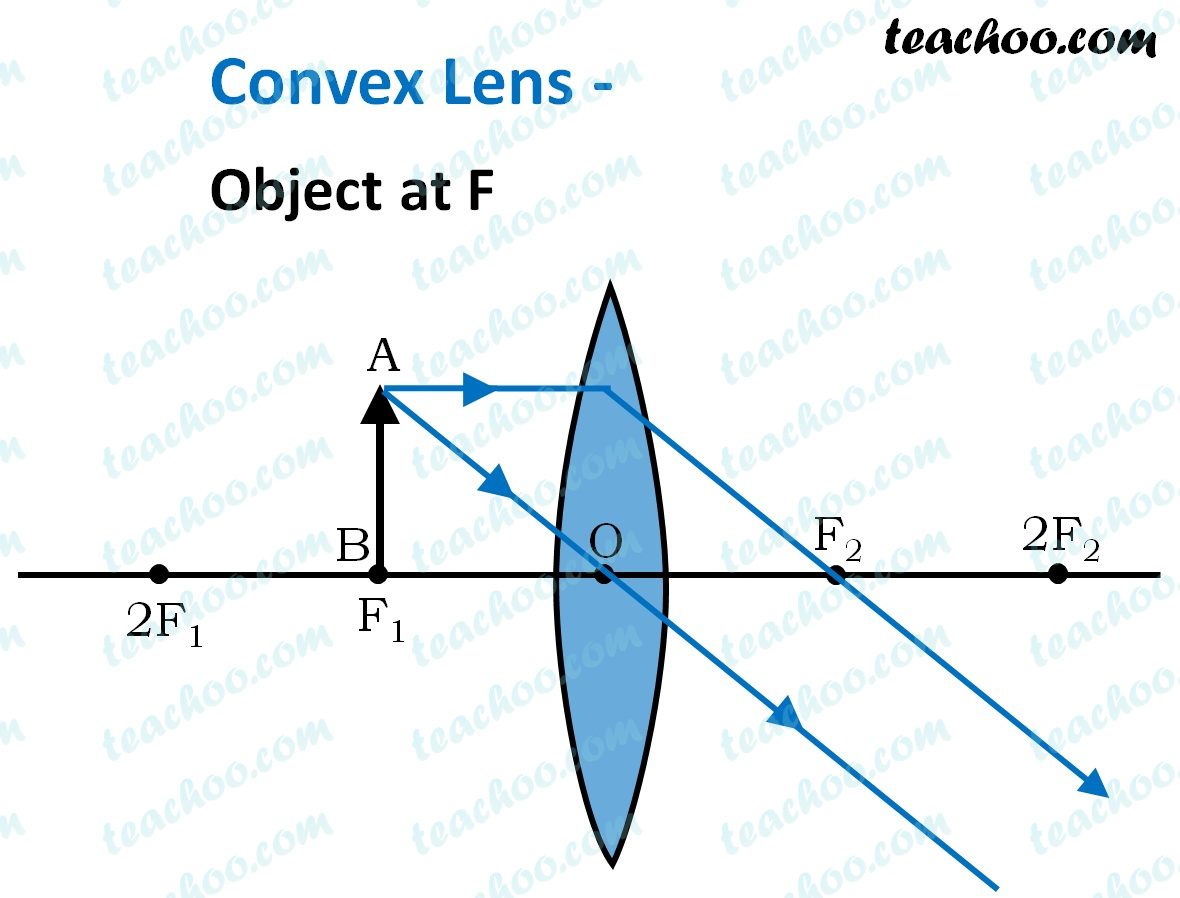Thus the correct answer is (iv) no image

Get live Maths 1-on-1 Classs - Class 6 to 12